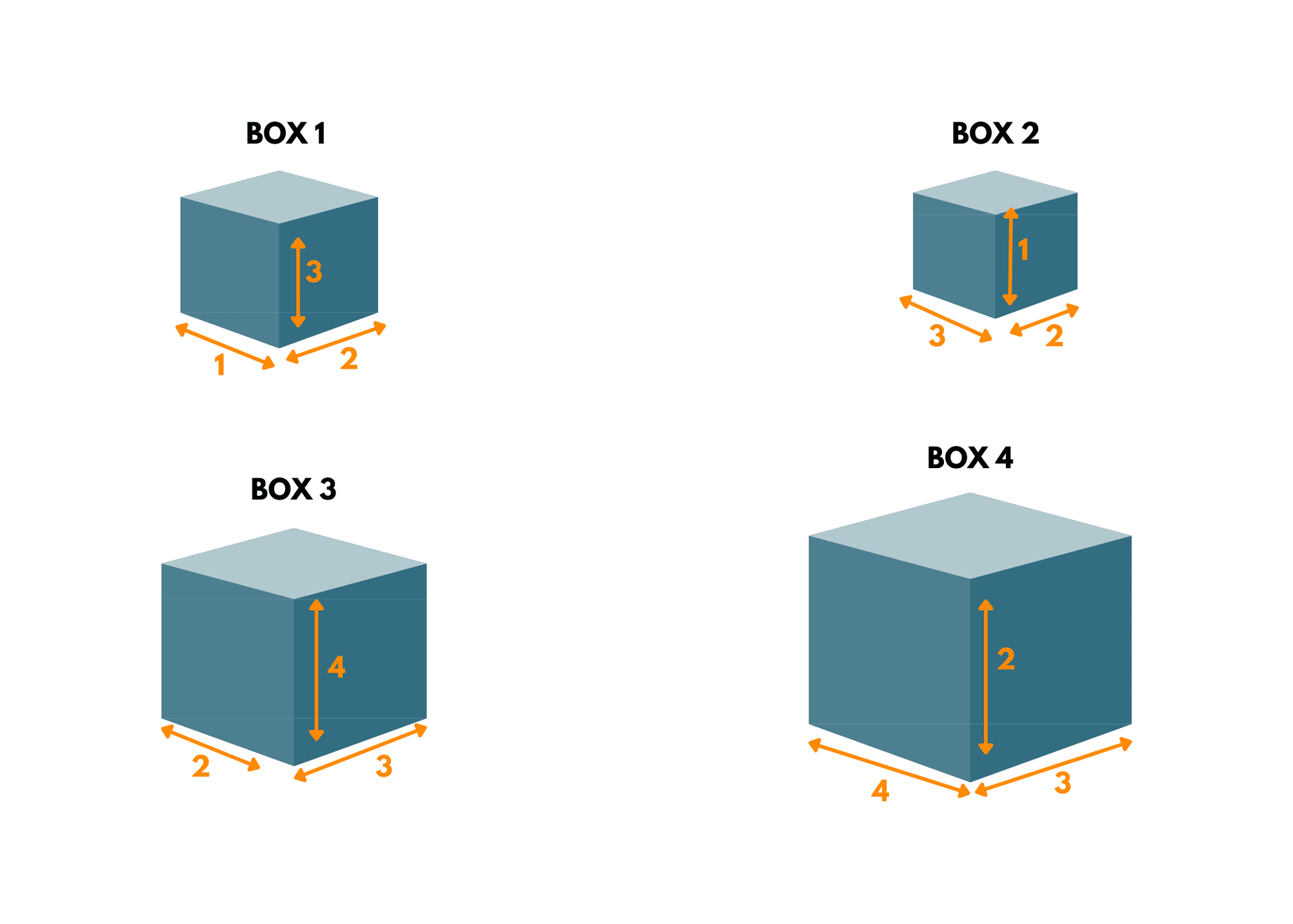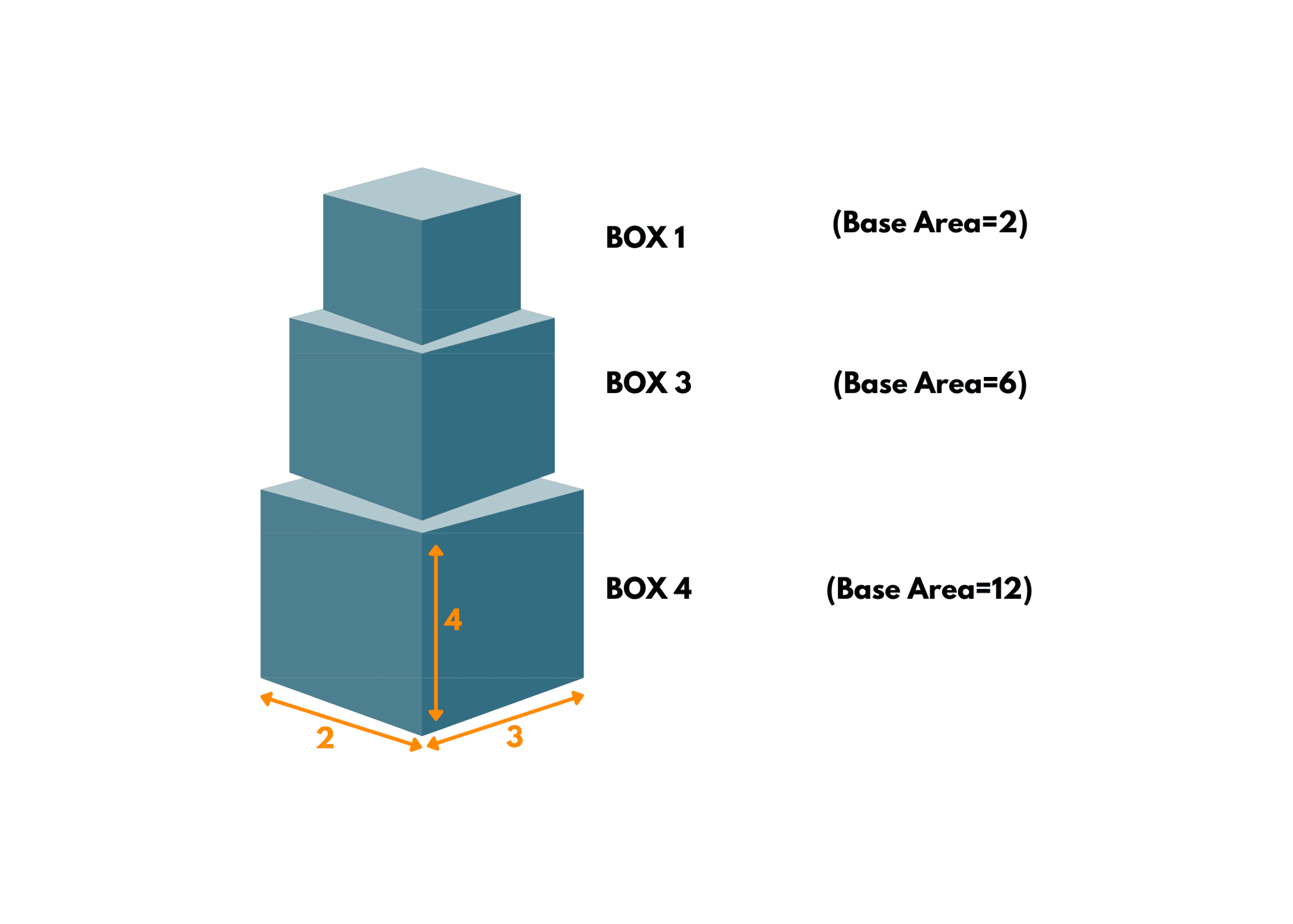# Box Stacking Problem (with C++ code)

Box Stacking is a classic computational challenge that involves arranging a set of boxes in a stable and space-efficient manner. In this article, we will learn more about the Box Stacking Problem and various methods to solve it.

## What is the Box Stacking Problem?

The objective of The Box Stacking Problem is to stack the boxes in such a way that the total height of the stack is maximized while adhering to certain constraints. This problem finds applications in various fields, such as logistics, warehousing, and container loading, where efficient space utilization is crucial.

Here is the problem statement of the problem: Given a set of rectangular boxes with different dimensions, our goal is to create a stable stack of maximum height by arranging the boxes one on top of another.

The constraints to be considered include the requirement that a box can only be placed on top of another if its base dimensions i.e. the length and width are smaller, and the boxes can only be rotated in one direction (e.g., 90 degrees). Additionally, each box can be used multiple times if available.

Also, this problem belongs to the class of NP-hard, which means that there is no known polynomial-time algorithm to solve it optimally for all cases. As the number of boxes increases, the problem becomes increasingly difficult to solve in a reasonable amount of time. Therefore, various heuristics and approximation algorithms have been developed to find near-optimal solutions efficiently.

### Box Stacking Example

Suppose we have the following set of boxes, each represented by their dimensions (length, width, height):

Box 1: (1, 2, 3)

Box 2: (3, 2, 1)

Box 3: (2, 3, 4)

Box 4: (4, 3, 2)Step 1: Generate All Possible Rotations For each box, we generate all possible rotations. Since each box has three dimensions, we get three rotations for each box:

Rotated Boxes: Box 1: (1, 2, 3) -> Box 1: (1, 2, 3) Box 1: (2, 1, 3) Box 1: (3, 1, 2)

Box 2: (3, 2, 1) -> Box 2: (3, 2, 1) Box 2: (2, 3, 1) Box 2: (1, 3, 2)

Box 3: (2, 3, 4) -> Box 3: (2, 3, 4) Box 3: (3, 2, 4) Box 3: (4, 2, 3)

Box 4: (4, 3, 2) -> Box 4: (4, 3, 2) Box 4: (3, 4, 2) Box 4: (2, 4, 3)

Step 2: Sort the Boxes Now, we sort the rotated boxes in decreasing order of their base area (length * width):

Sorted Rotated Boxes: Box 3: (2, 3, 4) Box 4: (4, 3, 2) Box 1: (1, 2, 3) Box 2: (3, 2, 1)

Step 3: Dynamic Programming Approach We use dynamic programming to find the maximum height achievable by box stacking. We create an array called "maxHeight" to store the maximum height achievable for each box when it is placed at the top of the stack. We initialize this array with the heights of each box.

maxHeight = [4, 2, 3, 1]

Step 4: Calculate Max Height Starting from the second box (Box 4), we check if each box can be placed on top of any of the boxes before it (Box 3, Box 4, and Box 1). We update the "maxHeight" value for each box as the maximum height achievable by placing it on top of that box plus its own height.

maxHeight = [4, 6, 7, 4]

Step 5: Find the Maximum Height After iterating through all the boxes and updating their "maxHeight" values, the maximum value in the "maxHeight" array will be the maximum height achievable by box stacking, which is 7.

Final Result: The maximum height achievable by stacking the given set of boxes is 7 units.Illustratively, the boxes are stacked as follows:

1. Box 3 (2, 3, 4) at the bottom
2. Box 1 (1, 2, 3) on top of Box 3
3. Box 2 (3, 2, 1) on top of Box 1
4. Box 4 (4, 3, 2) on top of Box 2

This arrangement yields a maximum height of 7 units.

### Algorithm to Solve The Problem

Here's a step-by-step algorithm to solve the box stacking problem using dynamic programming:

```boxStacking(boxes):
rotatedBoxes = generateAllRotations(boxes)
sort(rotatedBoxes, by base area in descending order)

n = length of rotatedBoxes
maxHeight = array of size n initialized with heights of boxes

for i from 1 to n-1:
for j from 0 to i-1:
if rotatedBoxes[i] can be placed on top of rotatedBoxes[j]:
maxHeight[i] = max(maxHeight[i], maxHeight[j] + rotatedBoxes[i].height)

maxStackingHeight = max value in maxHeight
return maxStackingHeight
```

This algorithm ensures that the solution is found efficiently by avoiding redundant calculations through dynamic programming. Sorting the boxes based on their base area also helps optimize the process of selecting the boxes to stack.

### Strategies for Solving the Box-Stacking Problem

1. Dynamic Programming: One approach to solving the box stacking problem is by employing dynamic programming techniques. This method involves breaking down the problem into smaller subproblems and solving them iteratively. By calculating the maximum height achievable for each subproblem, we can gradually build up the solution for the entire stack. However, dynamic programming has limitations in terms of the problem's input size, as the number of subproblems can grow exponentially.

2. Bottom-Up Greedy Algorithm: Another commonly used strategy is the bottom-up greedy algorithm. This algorithm sorts the boxes based on their base area in decreasing order. Starting from the largest box, it iteratively attempts to place each box on top of the existing stack, checking for stability and maximizing the height. This approach can provide a reasonably good solution, but it does not guarantee the optimal arrangement in all cases.

3. Modified Greedy Algorithm: A modification to the greedy algorithm involves considering all possible rotations of each box. By evaluating the stability and height of the stack for each rotation, we can select the most favorable placement. This modification increases the search space but allows for better utilization of the available boxes.

## Implementation of the Box Stacking Problem

Here's the C++ implementation for the box stacking problem:

```#include <iostream>
#include <vector>
#include <algorithms>

using namespace std;

struct Box {
int length, width, height;
Box(int l, int w, int h) : length(l), width(w), height(h) {}
};

// Compare function to sort boxes in decreasing order of base area
bool compare(Box& box1, Box& box2) {
return (box1.length * box1.width) > (box2.length * box2.width);
}

// Function to find the maximum height achievable by box stacking
int maxHeightBoxStacking(vector<Box>& boxes) {
// Generate all possible rotations of each box to create more options
vector<Box> rotatedBoxes;
for (const auto& box : boxes) {
rotatedBoxes.push_back(box);
rotatedBoxes.push_back(Box(box.width, box.height, box.length));
rotatedBoxes.push_back(Box(box.height, box.length, box.width));
}

// Sort the boxes in decreasing order of base area
sort(rotatedBoxes.begin(), rotatedBoxes.end(), compare);

int n = rotatedBoxes.size();
vector<int> maxHeight(n, 0);

for (int i = 0; i < n; ++i) {
maxHeight[i] = rotatedBoxes[i].height;
for (int j = 0; j < i; ++j) {
if (rotatedBoxes[i].length < rotatedBoxes[j].length &&
rotatedBoxes[i].width < rotatedBoxes[j].width) {
maxHeight[i] = max(maxHeight[i], maxHeight[j] + rotatedBoxes[i].height);
}
}
}

// Find the maximum height among all possible box stackings
int maxStackingHeight = 0;
for (int i = 0; i < n; ++i) {
maxStackingHeight = max(maxStackingHeight, maxHeight[i]);
}

return maxStackingHeight;
}

int main() {
// Example usage
vector<Box> boxes = {
{1, 2, 3},
{3, 2, 1},
{2, 3, 4},
{4, 3, 2}
};

int maxStackingHeight = maxHeightBoxStacking(boxes);
cout << "Maximum height achievable by box stacking: " << maxStackingHeight << endl;

return 0;
}
```

Output:

`Maximum height achievable by box stacking: 9`

The input consists of four boxes with dimensions:

• Box 1: 1 x 2 x 3
• Box 2: 3 x 2 x 1
• Box 3: 2 x 3 x 4
• Box 4: 4 x 3 x 2

The code calculates the maximum height achievable by stacking these boxes on top of each other, subject to the constraint that a box can only be placed on top of another box if its base area is smaller.

By placing Box 3 on top of Box 4, and then Box 1 on top of Box 3, we get the following stacking arrangement:

1. Box 4: 4 x 3 x 2
2. Box 3: 2 x 3 x 4
3. Box 1: 1 x 2 x 3

The total height achieved by this stacking is 2 + 4 + 3 = 9 units, which is the maximum height achievable by box stacking with the given set of boxes. Hence, the output is "Maximum height achievable by box stacking: 9".

Time Complexity: O(N * log(N))

• N is the number of boxes.
• The dominant factor in the time complexity is the sorting step that takes O(N * log(N)) time.

Space Complexity: O(N)

• N is the number of boxes.
• The algorithm uses additional space to store the rotated boxes and the maxHeight array, both of which have a space complexity of O(N).

## 2D Box-Stacking Problem

The 2D box stacking problem is a variation of the box stacking problem, but with a constraint that the boxes can only be stacked in two dimensions (without rotating them) instead of the three dimensions considered in the standard box stacking problem.

It is usually easier to solve than the standard 3D box stacking problem because there are fewer possible configurations to consider due to the absence of rotations. It can be solved efficiently using dynamic programming or other optimization techniques.

The goal is to maximize the total height achieved while adhering to the 2D stacking constraint.

## Conclusion

The box stacking problem presents a challenging task in optimizing space utilization and stack stability. Researchers continue to explore innovative techniques to tackle this problem and enhance the efficiency of box stacking in real-world applications.

### FavTutor - 24x7 Live Coding Help from Expert Tutors!